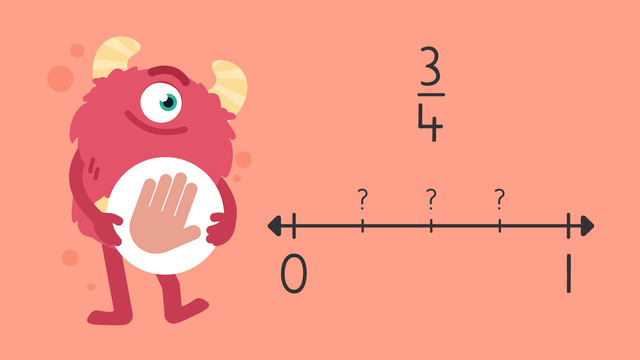# Fractions on a Number Line — Let's Practice!Rating

Ø 3.7 / 6 ratings
The authorsTeam Digital
Fractions on a Number Line — Let's Practice!
CCSS.MATH.CONTENT.3.NF.A.2.A CCSS.MATH.CONTENT.3.NF.A.2.B

## Basics on the topicFractions on a Number Line — Let's Practice!

Today we are practicing fractions on a number line with Razzi! This video contains examples to help you further practice and grow confident in this topic.

### TranscriptFractions on a Number Line — Let's Practice!

Razzi says get these items ready, because today we're going to practice fractions on a number line. It's time to begin! Use the number line to plot one half. Pause the video to work on the problem, and press play when you are ready to see the solution! Divide the number line into two parts matching the denominator, and count forward one part for the numerator. One half goes here! Did you also label one half here? Let's tackle the next problem! Use the number line to plot three fourths. Pause the video to work on the problem, and press play when you are ready to see the solution! Divide the number line into four parts matching the denominator, and count forward three parts for the numerator. Three fourths goes here! Did you also label three fourths here? Let's tackle the final problem! Use the number line to plot three thirds. Pause the video to work on the problem, and press play when you are ready to see the solution! Divide the number line into three parts matching the denominator, and count forward three parts for the numerator. Three thirds goes here! Three thirds is the same as one whole! Did you also label three thirds here? Razzi had so much fun practicing with you today! See you next time!